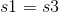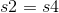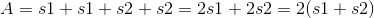## Example Questions

### Example Question #4 : Quadrilaterals

Find the area of a parallelogram with length 6 and height 5.Explanation:

To solve, simply use the formula for the area of a parallelogram. Thus,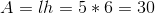Don't let a parallelogram fool you. It is just like a rectangle but on a slant. As long as you are given the height, and not the slant side length, you can use the same formula.

### Example Question #5 : Quadrilaterals

Find the perimeter of a parallelogram with one side equal to 6 and the other to 5.Explanation:

To solve, simply use the formula for the perimeter of a parallelogram. Thus,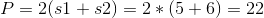If you don't remember this formula, you can also just sum up all the sides of the parallelogram.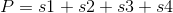Where s1 and s3 are the long sides and s2 and s4 are the short sides. Since the two pairs of sides are equal, you can sub in the following.